# NCERT Solutions For Class 10 Maths Chapter 1

### Download PDF of NCERT Solutions for Class 10 Maths Chapter 1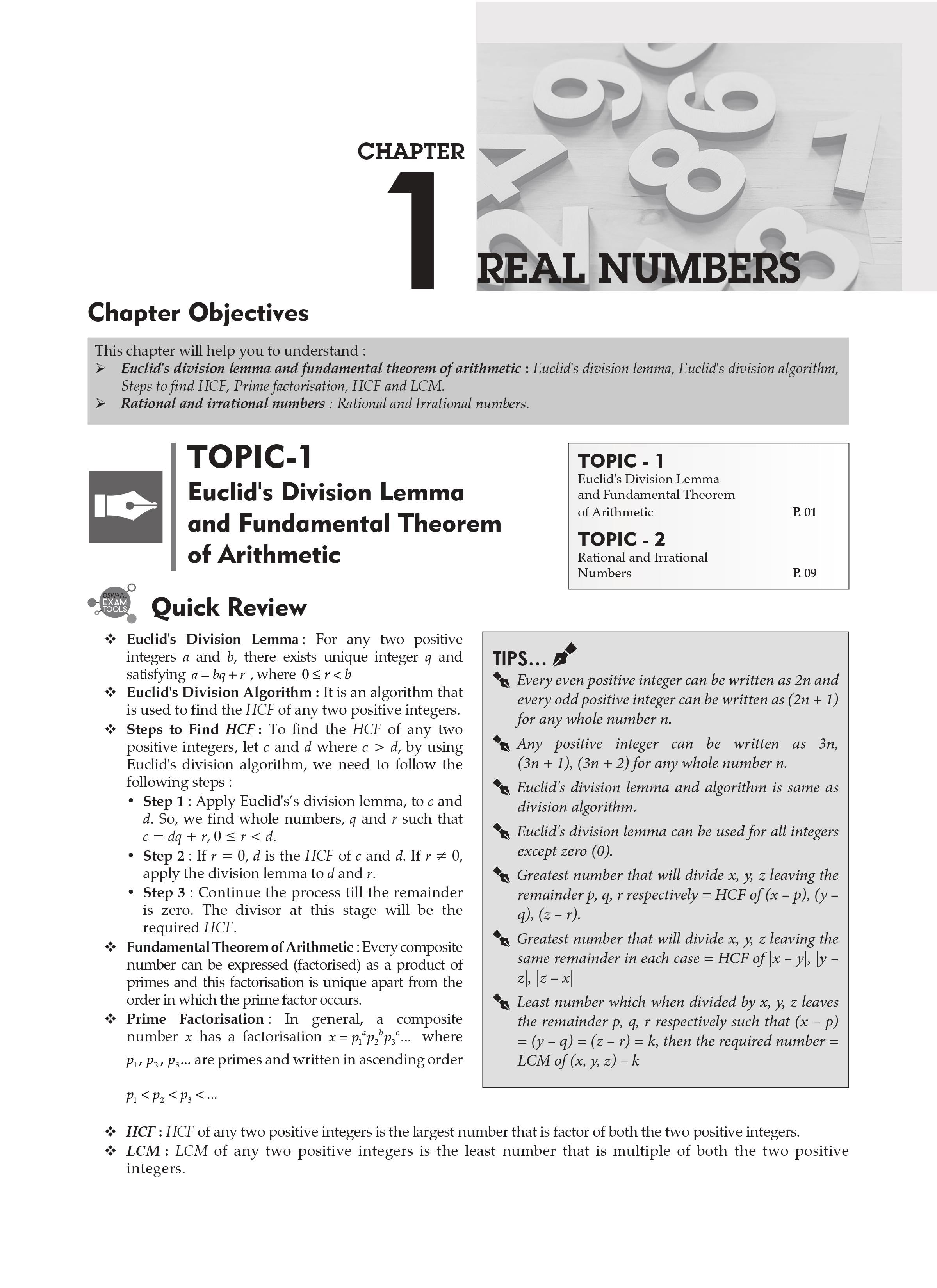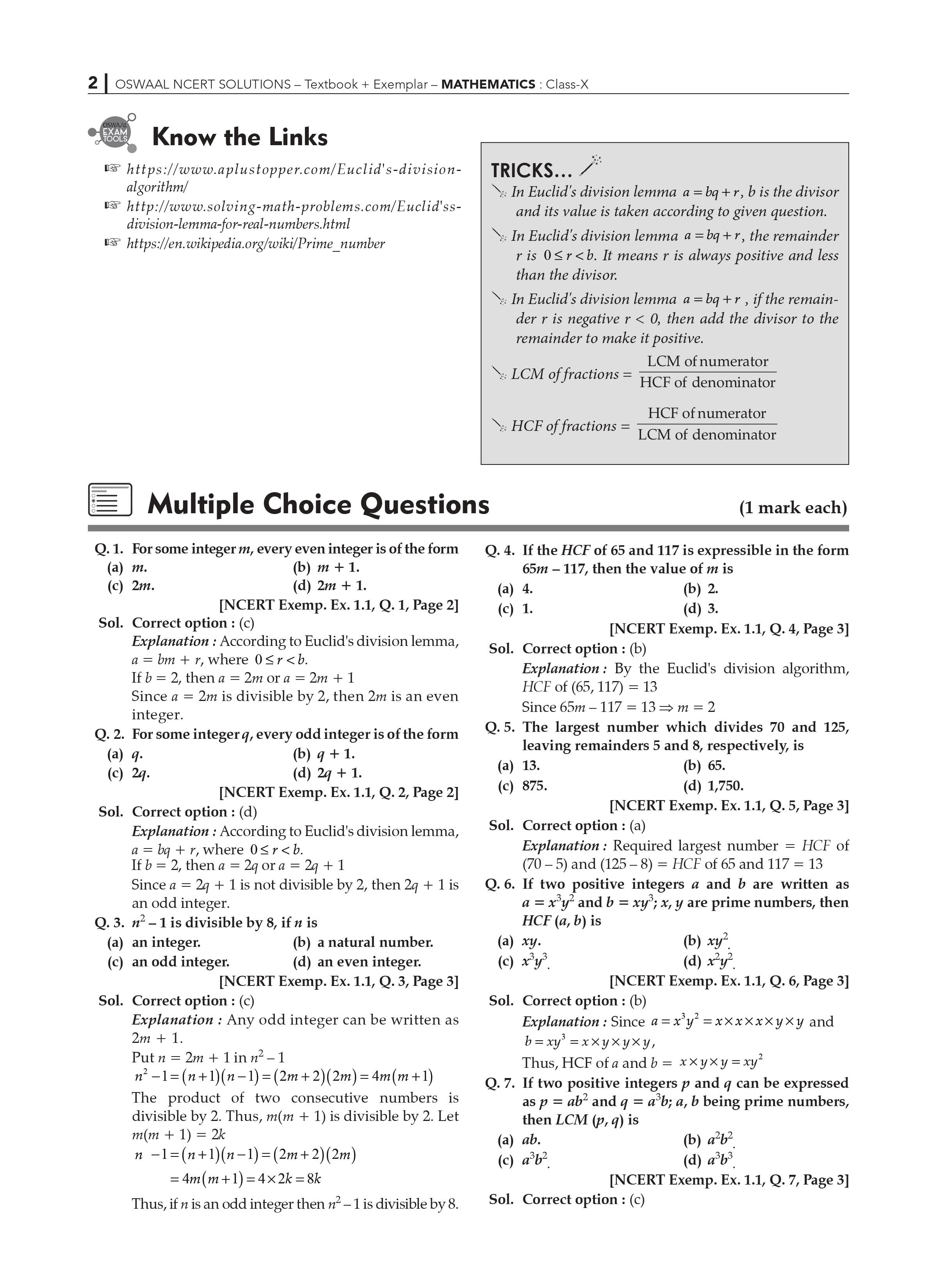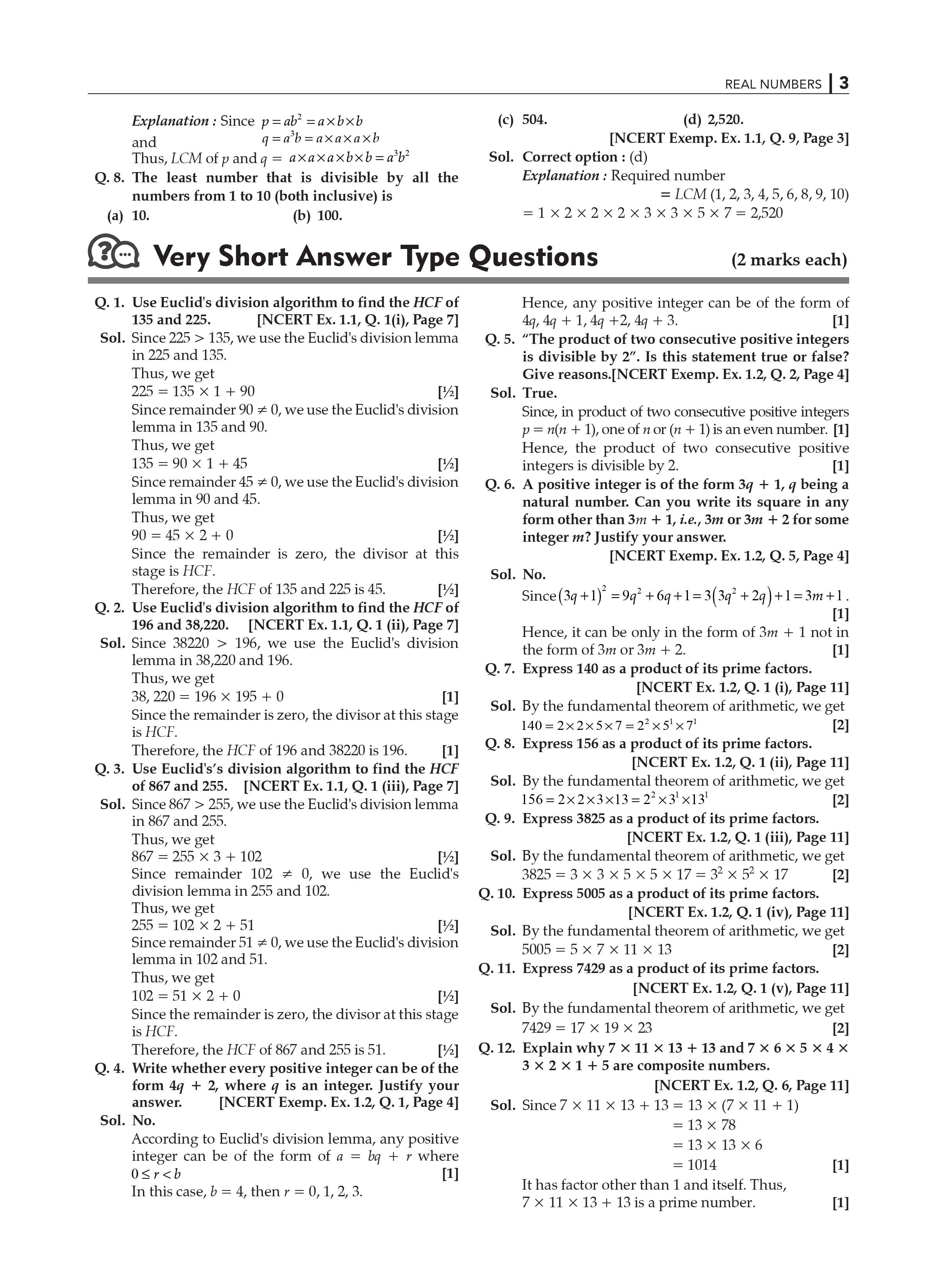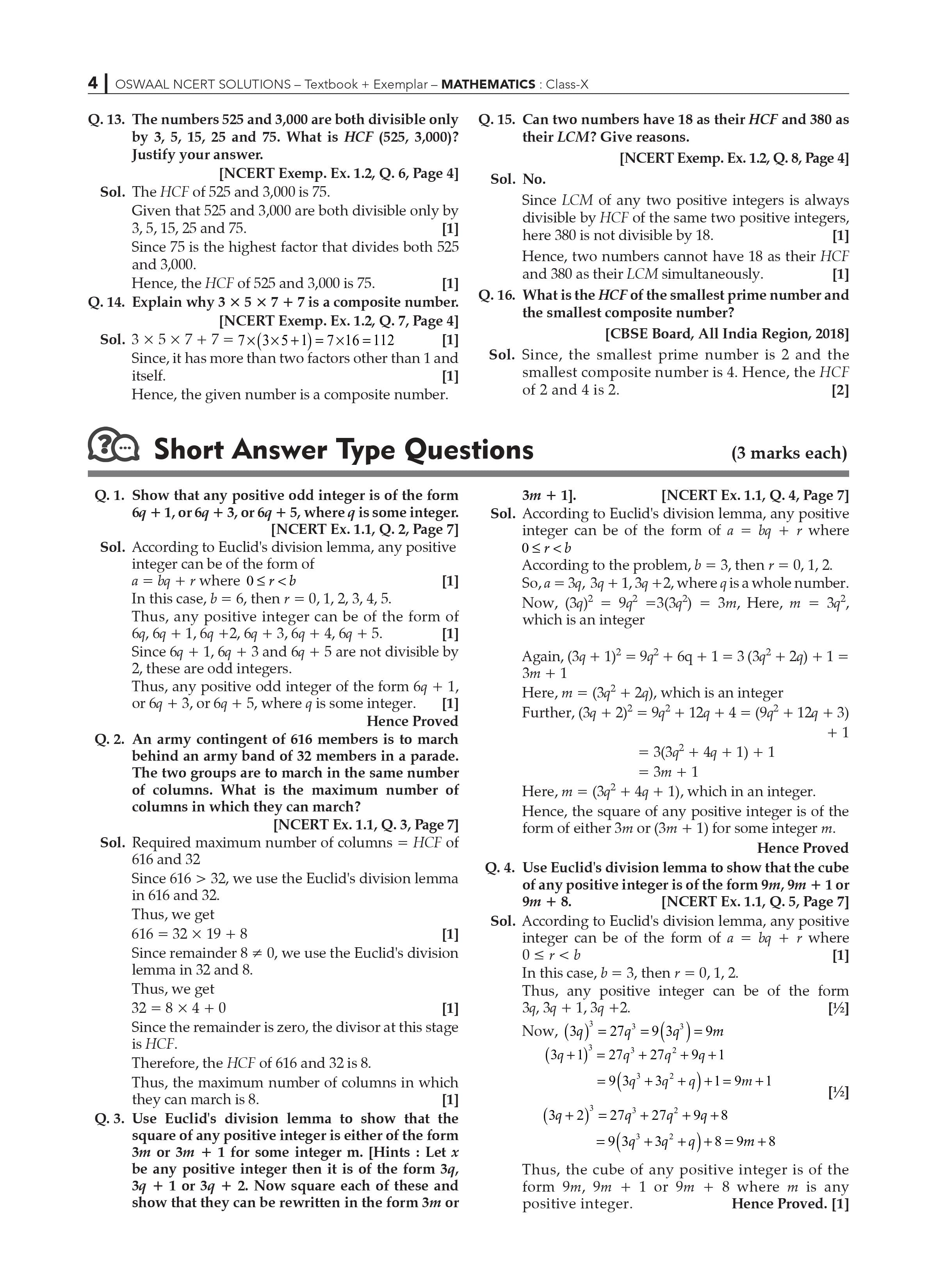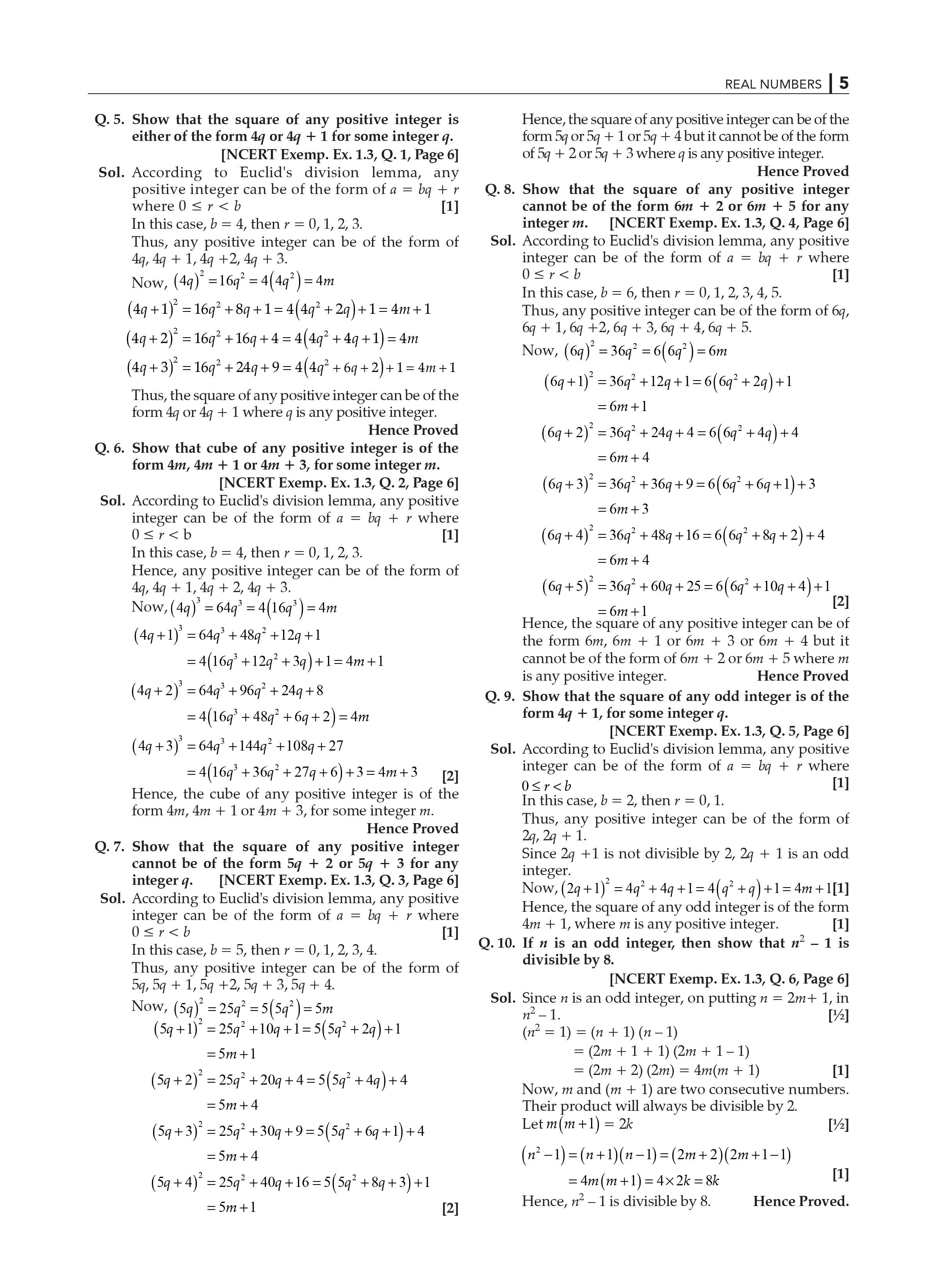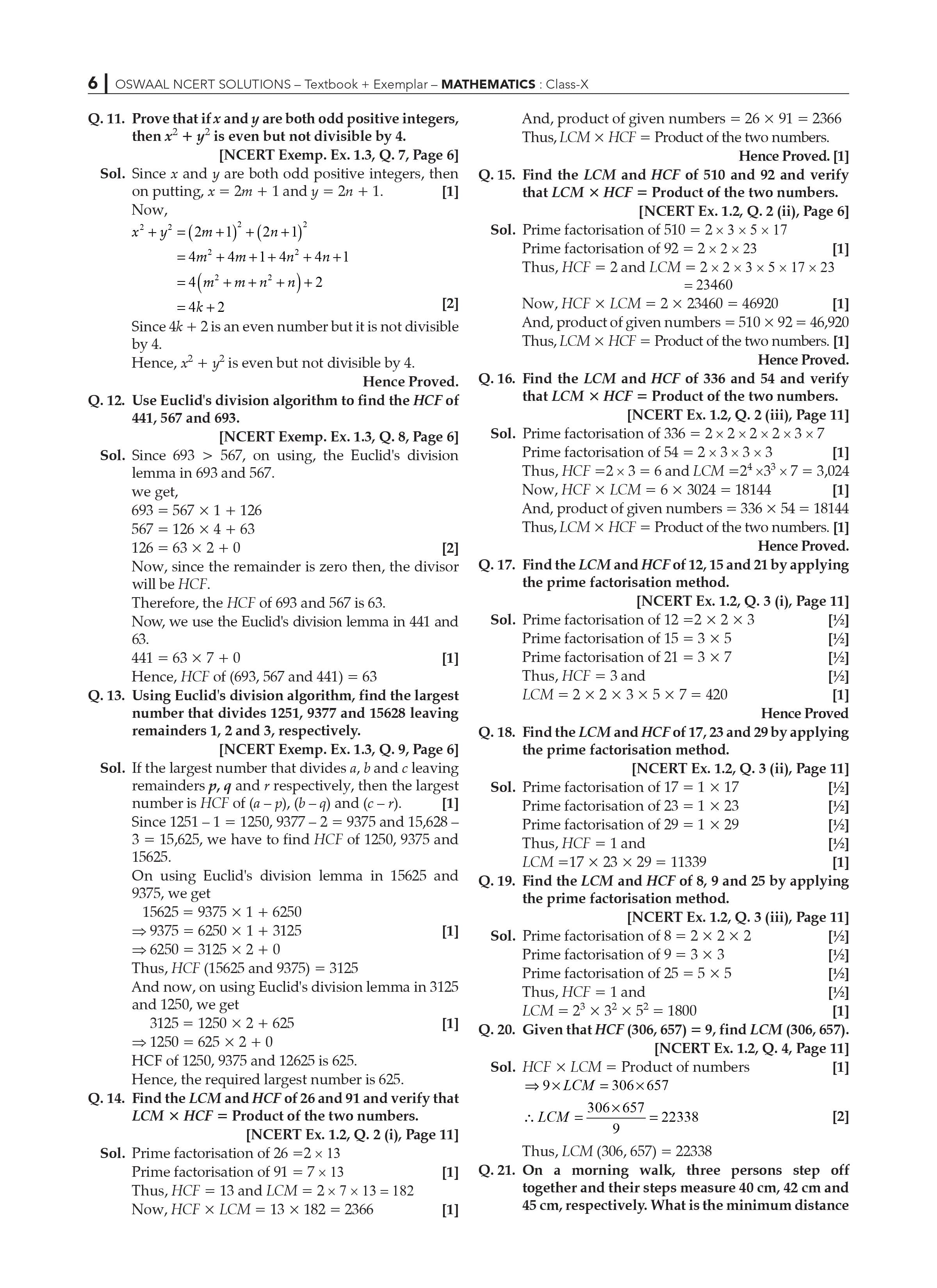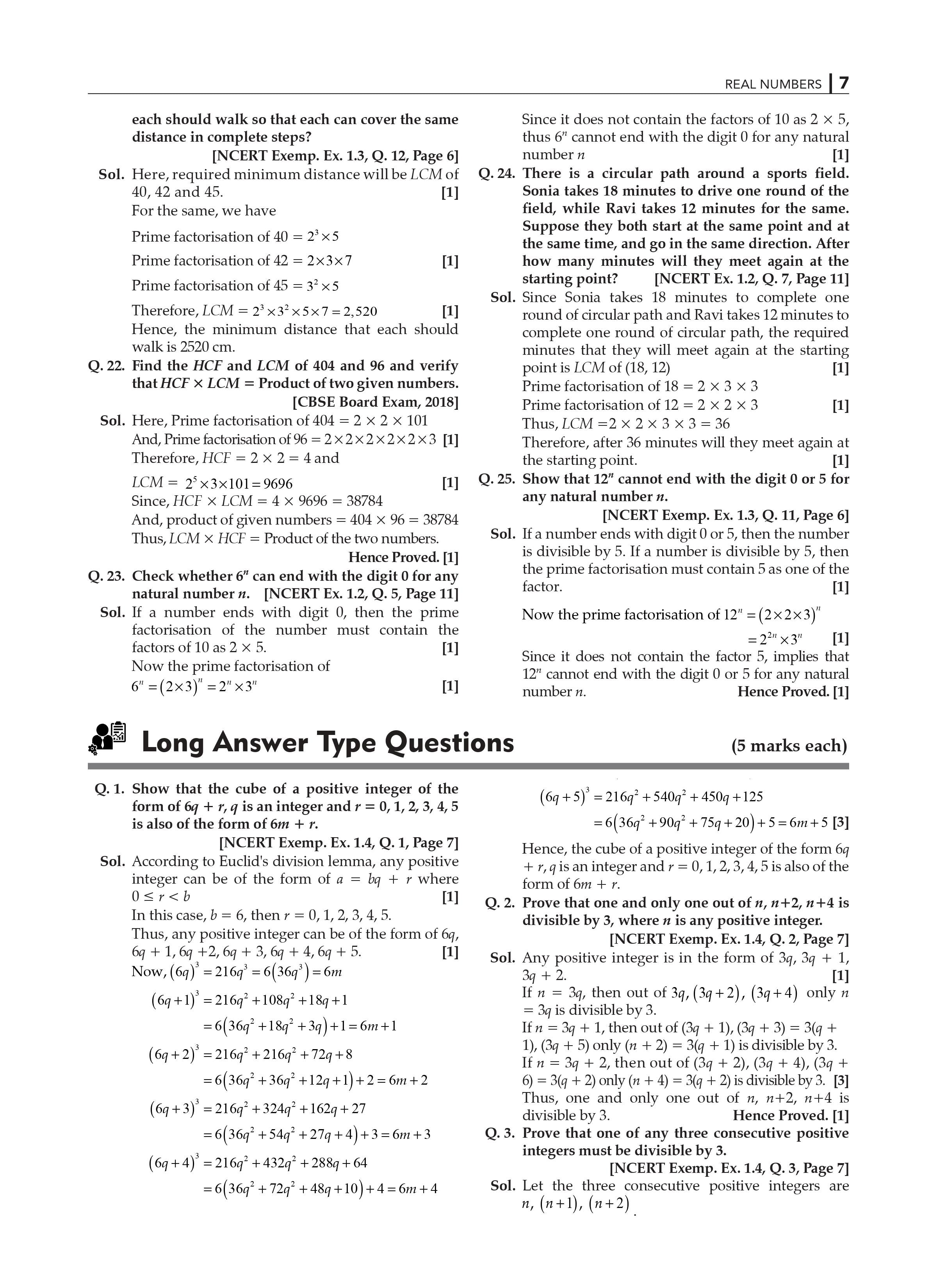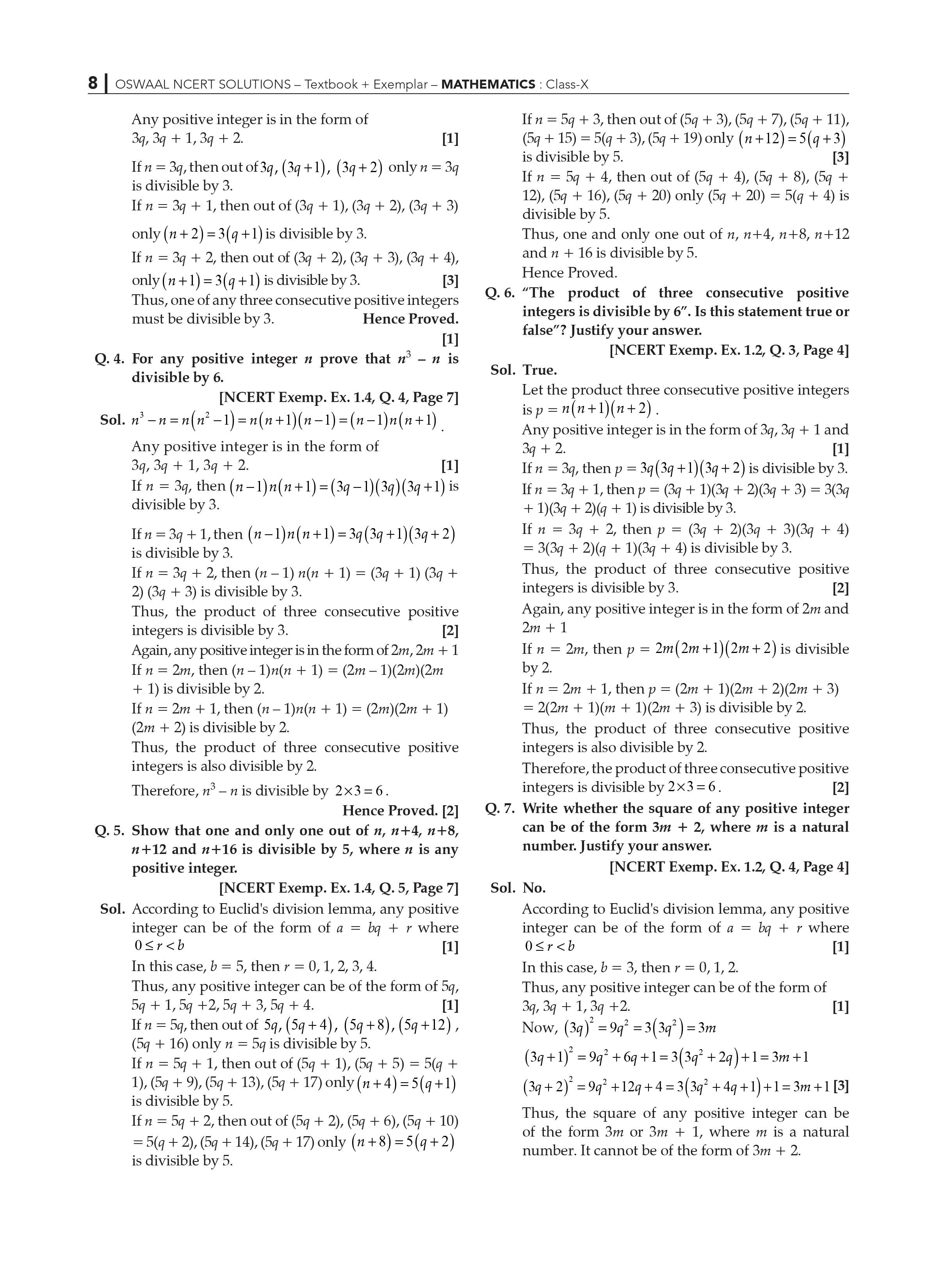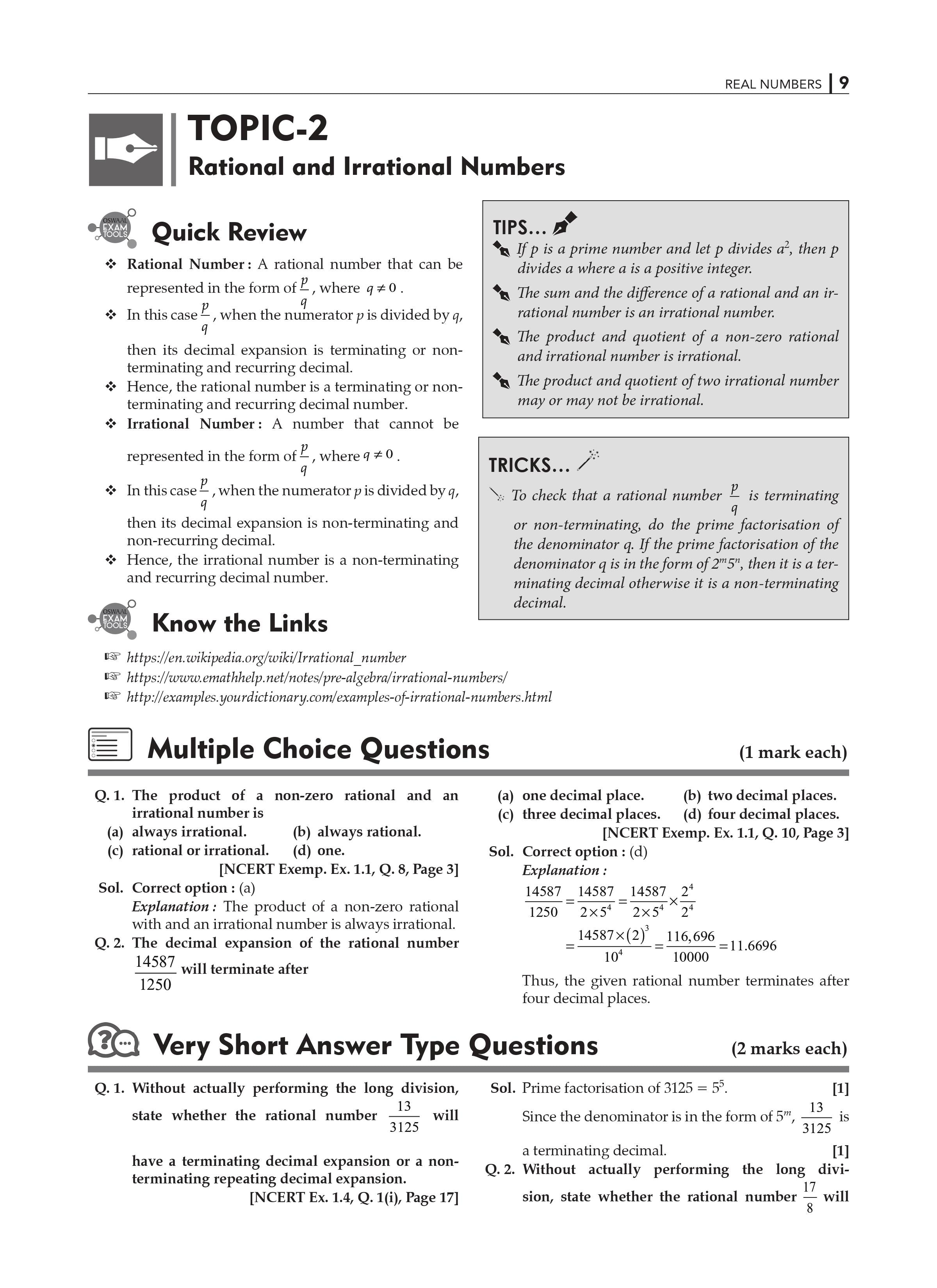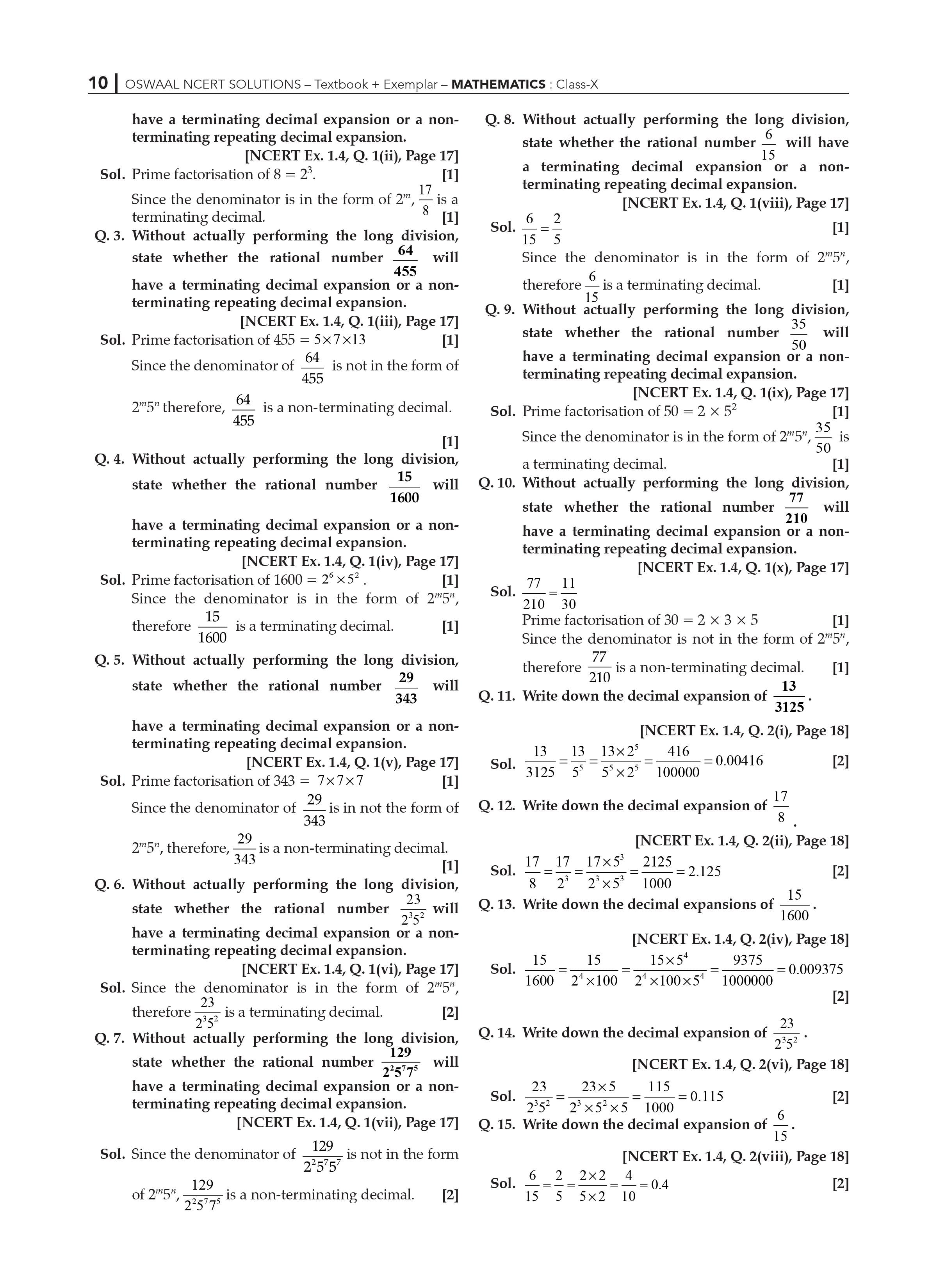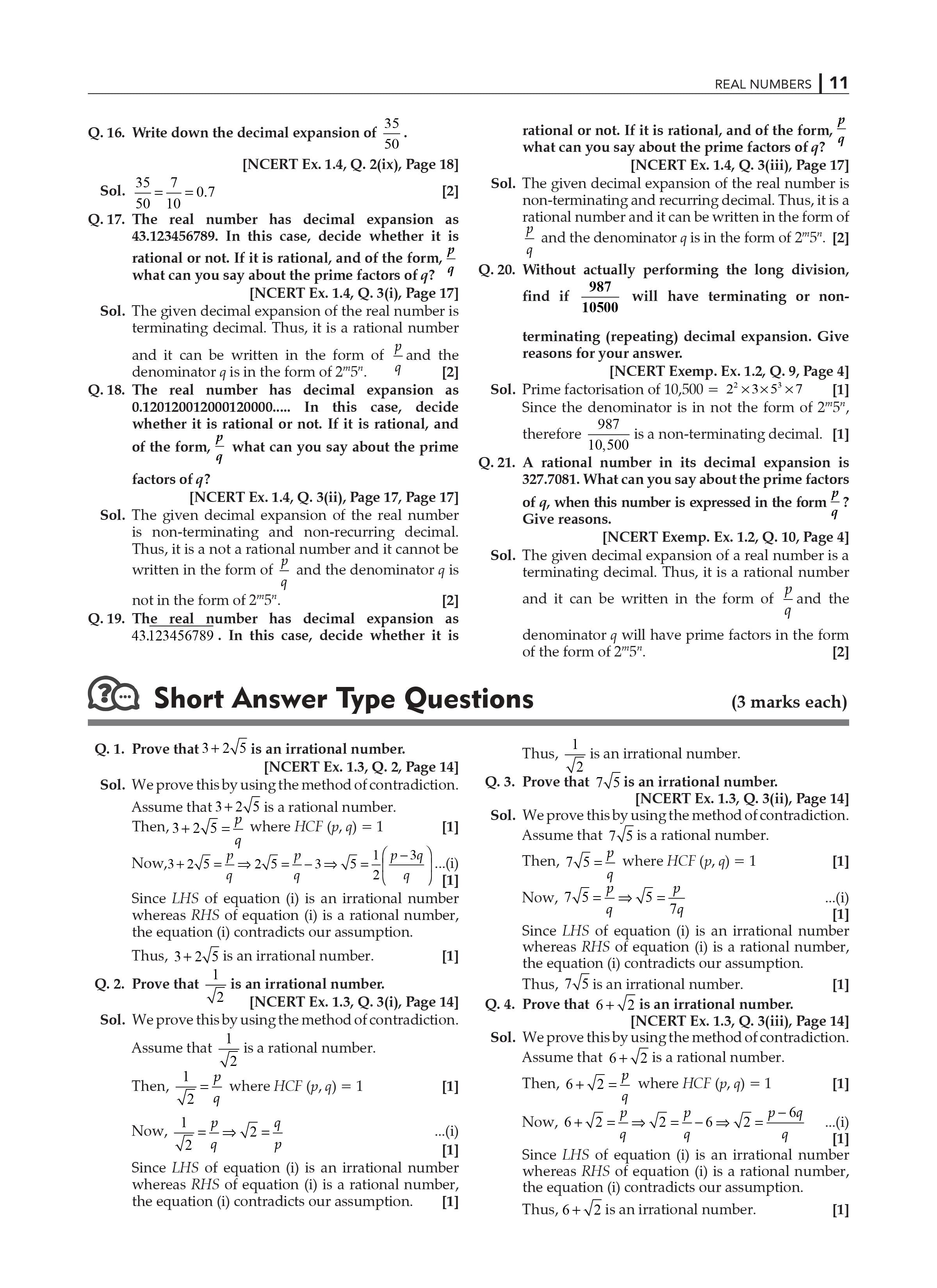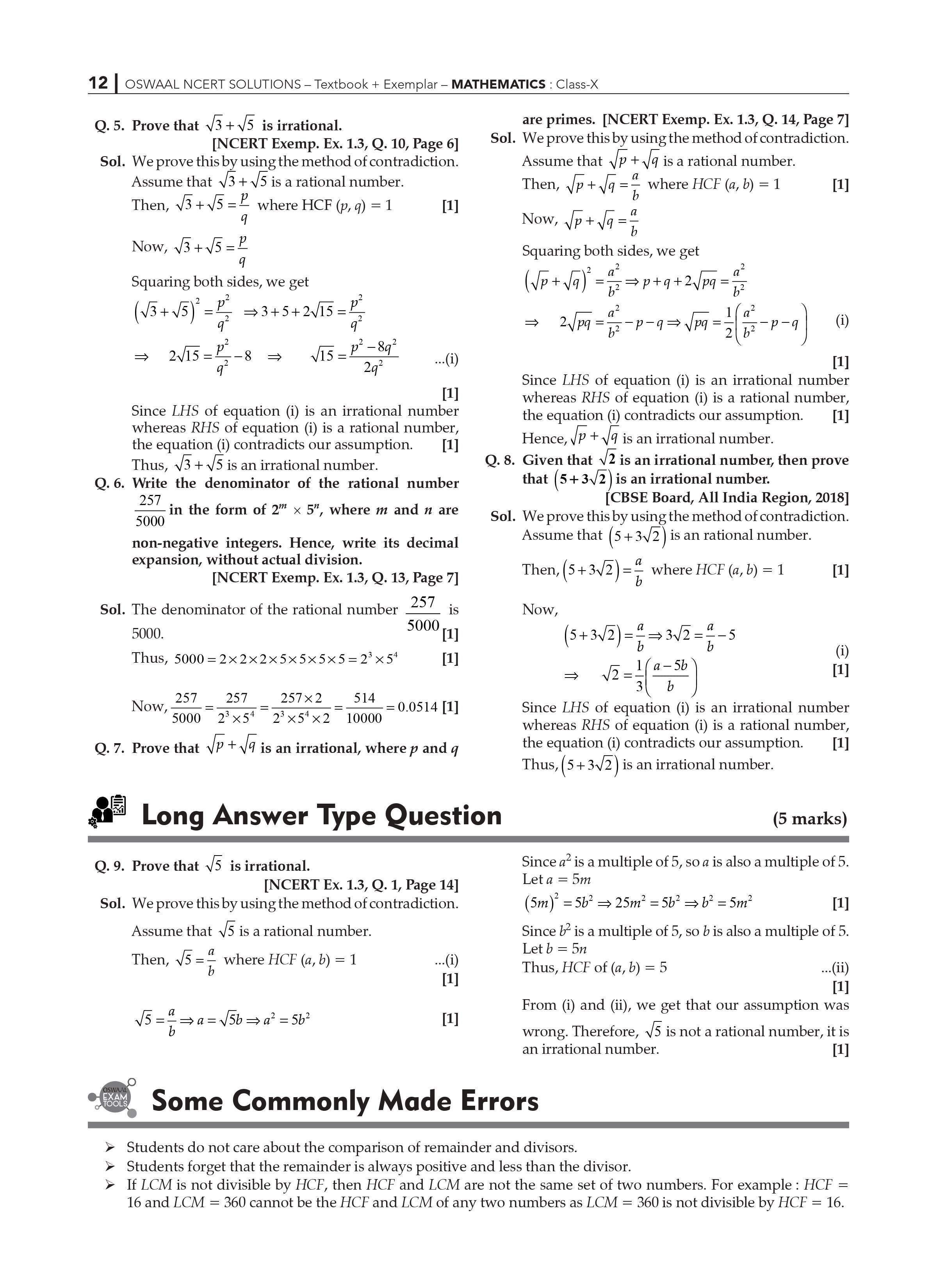Extra Information: NCERT Exemplar for Class 10 Maths Chapter 1 CBSE Notes for Class 10 Maths Chapter 1

NCERT Solutions for class 10 Maths Chapter 1- Real Number Exercise 1.1

Our subject-matter experts created these answers to assist students with their Class 10 2023 preparations. These experts go over each chapter of the NCERT Maths Solutions to make it easier for pupils to solve problems while utilizing it as a reference. They also put a lot of effort into making the NCERT Solutions for these exercises as simple as possible for the students.

The first exercise in Real Numbers, Exercise, uses Euclid's Division Algorithm to demonstrate the divisibility of integers. They provide a detailed and step-by-step explanation of each question's answer.

Presented in the NCERT textbook for the exercises of Class 10 Maths, the solutions are always created in accordance with NCERT criteria in order to cover the entire syllabus. These are really beneficial when it comes to getting good grades on tests.

NCERT solutions for class 10 Maths chapter 1: The first exercise in Chapter 1 of Class 10 Maths is Real Numbers.

Real numbers are introduced in Class 9 and explored in greater depth in Class 10 through the study of Euclid’s division algorithm.

The divisibility of numbers is discussed in this exercise. According to the divisibility of integers using Euclid’s division procedure, any positive integer a may be divided by another positive integer b with a remainder that is less than b.

NCERT Solutions for Class 10 Maths: Key Features Chapter 1: Real Number

• These NCERT Solutions will assist you in resolving and
• These NCERT Solutions will assist you in answering and revising all of the questions in Exercise 1.1.
• You will be able to obtain higher grades after going through the step-by-step solutions provided by our subject specialist lecturers.
• It aids exam success in the subject of mathematics.
• It adheres to NCERT criteria, which aid in the proper preparation of pupils.
• From the standpoint of the examination, it comprises all of the key questions.

NCERT Solutions for Class 10 Maths Exercise 1.2 Chapter 1 Real Numbers:

Here are the NCERT Solutions for Class 10 Maths Chapter 1 Real Numbers Exercise 1.2. These solutions are prepared by our subject experts with the goal of assisting students taking the CBSE 2023 exams. They prepare NCERT Math solutions for class 10 – chapter by chapter in order to assist students in swiftly solving issues.

The following considerations were made when generating these solutions: ease of comprehension and detailed description of the solutions presented.

• The fundamental theorem of Arithmetic is discussed in the exercise 1.2, which is the second exercise of Chapter 1 - Real numbers.
• In NCERT Exercise 1.2 Class 10, there are seven issues to solve, and solutions to all of them can be found here It is ensured that the offered solutions adhere to NCERT
• These solutions cover all of the concepts in this chapter, including real numbers, and will aid in exam success.
• This may be found here It is ensured that the offered solutions adhere to NCERT These solutions cover all of the concepts in this chapter, including real numbers, and will aid in exam success. These NCERT Solutions can be used to answer and revise all of the questions in Exercise 1.2 Real Numbers.

Key Features of NCERT Solutions for Class 10 Maths Chapter 1- Real Number:

• If you practice it completely, it will help you score well in math in tests.
• It adheres to NCERT criteria, which aid in the proper preparation of pupils.
• From the standpoint of the examination, it comprises all of the key questions.

NCERT Solutions for Class 10 Maths Exercise 1.3 Chapter 1 Real Numbers

NCERT's recommendations, here you can find the NCERT Class 10 Maths Chapter 1 Real Numbers Exercise 1.3. Our subject specialists have developed these solutions for NCERT problems. As a result, these are a valuable resource for pupils taking their 2023 exams in Class 10. These professionals go over the NCERT Maths Solution for Class 10 in two ways: chapter-by-chapter and exercise-by-exercise, so that students can quickly solve the questions.

They prefer to write these NCERT Solutions for Class 10 in such a way that the kids can grasp them. Exercise 1.3 is the third exercise in Class 10 Chapter 1: Real Numbers. The most crucial.

The importance of irrational numbers is emphasized here. Before attempting Exercise 1.3, you must have a fundamental understanding of rational and irrational numbers. Following the NCERT principles, students can also practice how to solve an issue. The solutions shown here are similar.

NCERT solutions for class 10 Maths chapter 1 exercise 1.3 The third exercise in Chapter 1 of Class 10 Maths is Real Numbers. Class 9 introduces real numbers, which are then examined in greater depth in Class 10. To comprehend these solutions, you must have a basic understanding of the topic - irrational numbers.

The activity explains how to show that root p is irrational.

Revisiting Irrational Numbers – It has three questions based on the theorem, the third of which has three roots that must be proven to be irrational.

Chapter 1- Real Number: Key Features of NCERT Solutions for Class 10 Maths

• After going through the step-by-step solutions, you will be able to solve and revise all of the questions in Exercise 1.3.
• You will be able to achieve higher grades with the help of our subject-matter experts.
• Following NCERT guidelines, these solutions were created.
• It aids in getting good grades in math on exams.
• From the standpoint of the examination, it comprises all of the key questions.

NCERT Solutions for Class 10 Maths Exercise 1.4 Chapter 1 Real Numbers

NCERT's recommendations, here is Chapter 1 Real Numbers Exercise 1.4 for Class 10 Maths. These NCERT Maths Solutions for Class 10 are prepared by our own subject specialists. They also go over these solutions from time to time to make changes so that the students can understand them.

These solutions are simple to practice and aid in quick revision for pupils. This exercise, the fourth exercise in Chapter 1 Real numbers in class 10, delves deeper into rational numbers and their decimal expansion. The solutions are presented in a progressive manner to make it easier to understand. Not only that, but we've also concentrated on the following.

The fourth exercise in Chapter 1 of class 10 Maths.

Real Numbers are introduced in Class 9 and then examined in greater depth in Class 10. It explains rational numbers and their decimal expansions in this lesson. The exercise explains when a rational number’s decimal expansion is terminating and when it is non-terminating.

Rational Numbers and their Decimal Expansions: A Review – It consists of three questions, the first of which has ten parts and the third of which has three parts.

Chapter 1- Real Number Exercise 1.4: Key Features of NCERT Solutions for Class 10 Maths

These NCERT Solutions will assist you in answering and revising all of the problems in Exercise 1.4.

After going over these NCERT solutions, you’ll be able to solve problems with more confidence.

NCERT Maths Solutions are provided by the National Council of Educational Research and Training (NCERT)

It not only aids in the revision of concepts but also improves exam performance.

It adheres to NCERT criteria, which aid in the proper preparation of pupils.

From the standpoint of the examination, it comprises all of the key questions.

What are the Important points of this chapter?

The NCERT Solutions for Class 10 Math’s Real Numbers cover the following topics in depth:

for Math’s in Class 10 Euclid's division algorithm, the fundamental theorem of arithmetic, rational and irrational numbers, and decimal expansions are all covered in Real Numbers. Our teachers have effectively built the solutions based on these notions. Students can achieve good grades in class examinations and the Class 10 first term exam by emphasizing these themes.

These NCERT Math’s Class 10 Chapter 1 Solutions assist students in deftly and efficiently handling problems.

For Math’s in Class 10 Euclid's division algorithm, the fundamental theorem of arithmetic, rational and irrational numbers, and decimal expansions are all covered in Real Numbers. The expert has effectively built the solutions depend upon these notions. Students can achieve good grades in class examinations and the Class 10 first term exam by emphasizing these themes. These NCERT Math’s Class 10 Chapter 1 Solutions assist students in deftly and efficiently handling problems.

Is it plays an important role in study NCERT Solutions for Class 10 Math’s Chapter 1 for the exam?

Yes, the first chapter of NCERT Solutions for Class 10: Real Numbers is crucial in terms of test preparation. It includes both short and lengthy answer questions to help you improve your problem-solving skills. These solutions were developed by our specialist faculty to aid students in their examination preparation.